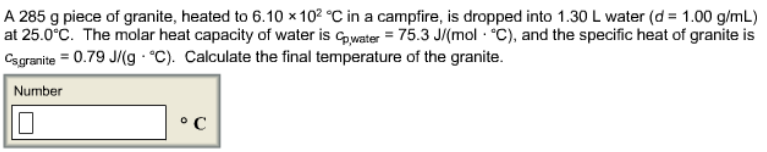# Problem: A 285 g piece of granite, heated to 6.10 x 102 °C in a campfire, is dropped into 1.30 L water (d = 1.00 g/mL) at 25.0 °C. The molar heat capacity of water is cp, water = 75.3 J/(mol • °C), and the specific heat of granite is Cs, granite = 0.79 J/(g • °C). Calculate the final temperature of the granite.

###### FREE Expert Solution
87% (425 ratings)###### Problem Details

A 285 g piece of granite, heated to 6.10 x 102 °C in a campfire, is dropped into 1.30 L water (d = 1.00 g/mL) at 25.0 °C. The molar heat capacity of water is cp, water = 75.3 J/(mol • °C), and the specific heat of granite is Cs, granite = 0.79 J/(g • °C). Calculate the final temperature of the granite.